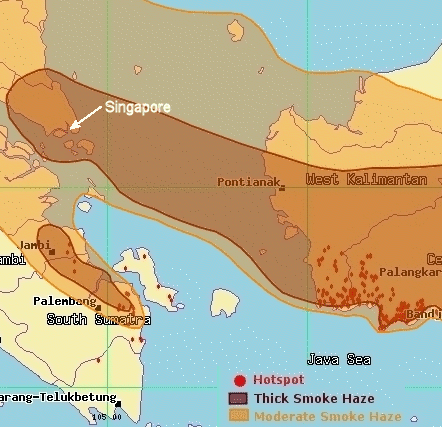Search IntMath
Close

# Escaping the haze

By Murray Bourne, 17 Oct 2006

It's starting to feel like 1997, the first year we were in Singapore. The haze was so bad then that we couldn't see more than a few hundred metres and we couldn't see the sun for several weeks.

Here's what it looked like at 4:00 pm yesterday:Source: Singapore's Environment Agency

I'm off to Bali where it is haze free. Woo hoo!

Be the first to comment below.

### Comment Preview

HTML: You can use simple tags like <b>, <a href="...">, etc.

To enter math, you can can either:

1. Use simple calculator-like input in the following format (surround your math in backticks, or qq on tablet or phone):
a^2 = sqrt(b^2 + c^2)
(See more on ASCIIMath syntax); or
2. Use simple LaTeX in the following format. Surround your math with $$ and $$.
$$\int g dx = \sqrt{\frac{a}{b}}$$
(This is standard simple LaTeX.)

NOTE: You can mix both types of math entry in your comment.